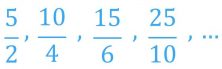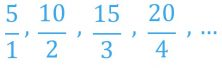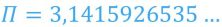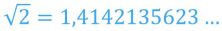Smartick is an advanced online program that teaches kids math and coding in only 15 min. a day

Oct01

# Getting to Know Rational Numbers and Their Properties

In today’s post, we are going to learn a little more about rational numbers.

In an earlier post, we saw how to represent numbers on a number line and in another, what types of rational numbers exist.

Now we are going to get to know them in a bit more detail.

### Properties of Rational Numbers

Rational numbers are those that can be represented as a ratio of two integers. It is to say, that we can represent them as a fraction a/b, where a and b are integers and b is a number other than zero.

The term ”rational” comes from the word ratio, meaning part of a whole. (for example: ”We had a ratio of three per person.”)

Each rational number can be represented by infinite equivalent fractions. For example, the rational number 2.5 can be represented by the following fractions:And by all the fractions equivalent to these.

The set of all rational numbers is represented by the following symbol:Remember that any integer is also a rational number and can be represented as a ratio of two integers.

For example, the number 5 can be represented by the following fractions:This means that the set of integers is contained within the set of rational numbers. Mathematically we write it as:To place the numbers on a number line, or real numbers, there are numbers that cannot be represented as a ratio of two integers.

These numbers are called irrational numbers, and the most well known are these:### The Rational Numbers of Ancient Egypt

Rational numbers emerge with the need to distribute a quantity D in d parts, where D is not a multiple of d.

To calculate the amount that will be distributed to each part, you need to perform the operation D:d, which does not result in an integer because D is not a multiple of d.

To find the result of this operation, there are numbers that appear and are able to represent the form D/d,  but they are different from integers.

In Ancient Egypt, they already had made these types of ”parts of an integer” deals using almost exclusively unit fractions, that had 1 as the numerator. It is to say that we can represent 1/b as a fraction, where b is a positive integer.

These unit fractions were represented by hieroglyphics in the shape of an ”open mouth” that represented the vinculum (line in a fraction), and a numerical hieroglyphic written below, which represented the denominator of the fraction.

For example, to represent 1/4 they wrote it in the following way:Any non-unit fraction was represented as the sum of different unit fractions. Therefore, the sums of unit fractions are known as Egyptian fractions.

What did you think of this post? Would you like to get to know rational numbers better? If this is the case, share the post so that others can learn as well.